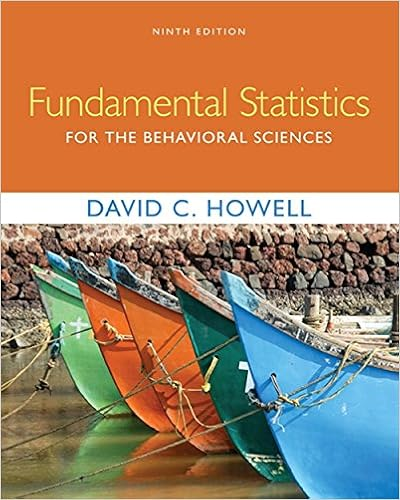# Stability she must also assume that the population of

• Lab Report
• 6

This preview shows page 1 - 4 out of 6 pages.

##### We have textbook solutions for you!
The document you are viewing contains questions related to this textbook.The document you are viewing contains questions related to this textbook.
Chapter 20 / Exercise E
Fundamental Statistics for the Behavioral Sciences
HowellExpert Verified
stability. She must also assume that the population of differences is normally distributed. Mackenzie can check this assumption by creating a QQ plot of those ten sample differences. Lab Workbook Page 53
##### We have textbook solutions for you!
The document you are viewing contains questions related to this textbook.The document you are viewing contains questions related to this textbook.
Chapter 20 / Exercise E
Fundamental Statistics for the Behavioral Sciences
HowellExpert Verified
The test statistic t is created by taking the sample mean difference and dividing by the standard error of the sample mean difference, 𝑡𝑡 = 𝑑𝑑 𝑠𝑠𝑠𝑠 ( 𝑑𝑑 ) If the null hypothesis is true, this test statistic has a t distribution with n -1 degrees of freedom, where n is the number of pairs. For Mackenzie’s 10 runners, the degrees of freedom will be 10 – 1 = 9. . Formula Card Warm-Up: Check Your Understanding Eight cars were run to determine their mileage, in miles per gallon (MPG). Then each car was given a tune- up, and run again to measure the mileage a second time. The difference in mileage was computed as difference = After MPG minus Before MPG. Assume that the selection of 8 cars represents a random sample of cars. 1. Select the appropriate alternative hypothesis to assess if on average mileage significantly improves after a tune up. 0
2. The researcher of course is hoping that the results of the experiment are statistically significant. What type of p -value would the researcher want to obtain?
Lab Workbook Page 54
ILP: Do Books Purchased from Barnes and Noble (In-store) Cost More on Average than If Purchased at Amazon.com (Online) ? Background: The popularity of purchasing books online has increased dramatically, and the conventional bookstore no longer dominates the sales of books. The most influential factor that sways customers into purchasing online is lower prices. A group of statistics students decided to perform a comparison of the Amazon.com prices versus local Barnes and Noble bookstore prices based on a sample of 40 books, selected from a wide range of categories. For Amazon, a standard ground shipping of \$4.29 and local state tax were included in the cost. The corresponding costs are available in the R data set called books.Rdata (Source: Statistics group project). Do the data provide sufficient evidence to conclude that, on average, Barnes and Noble (in-store) books are more expensive than Amazon.com books?
•••